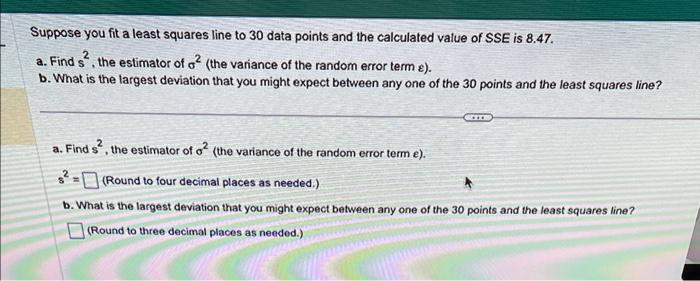Home / Expert Answers / Statistics and Probability / suppose-you-fit-a-least-squares-line-to-30-data-points-and-the-calculated-value-of-sse-is-8-47-pa298

# (Solved): Suppose you fit a least squares line to 30 data points and the calculated value of SSE is $$8.47 \ ...Suppose you fit a least squares line to 30 data points and the calculated value of SSE is \( 8.47$$. a. Find $$s^{2}$$, the estimator of $$\sigma^{2}$$ (the variance of the random error term $$\varepsilon$$ ). b. What is the largest deviation that you might expect between any one of the 30 points and the least squares line? a. Find $$s^{2}$$, the estimator of $$\sigma^{2}$$ (the variance of the random error term $$e$$ ). $s^{2}=\text { (Round to four decimal places as needed.) }$ b. What is the largest deviation that you might expect between any one of the 30 points and the least squares line? (Round to three decimal places as needed.)

We have an Answer from Expert